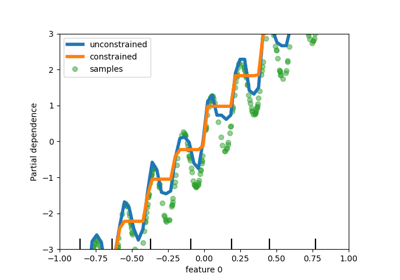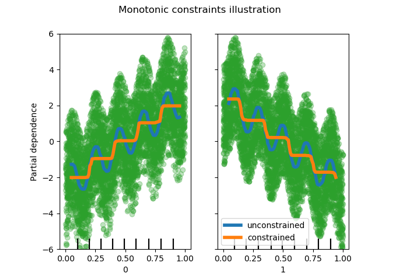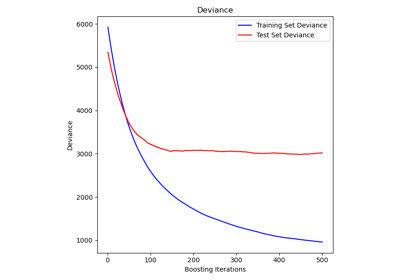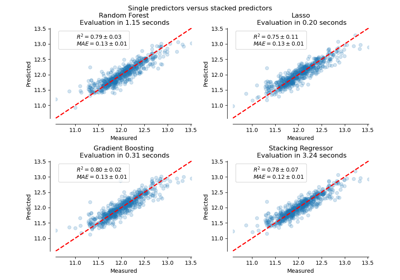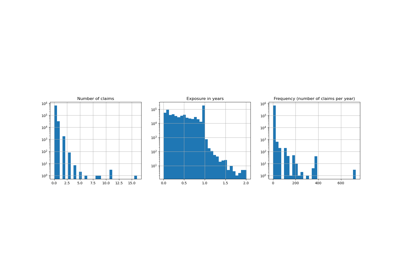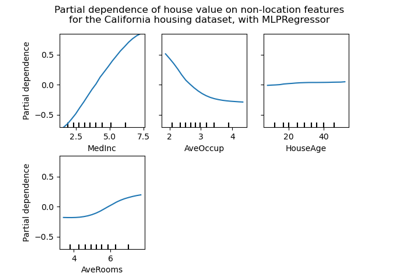# sklearn.ensemble.HistGradientBoostingRegressor¶

class sklearn.ensemble.HistGradientBoostingRegressor(loss='least_squares', *, learning_rate=0.1, max_iter=100, max_leaf_nodes=31, max_depth=None, min_samples_leaf=20, l2_regularization=0.0, max_bins=255, monotonic_cst=None, warm_start=False, early_stopping='auto', scoring='loss', validation_fraction=0.1, n_iter_no_change=10, tol=1e-07, verbose=0, random_state=None)[source]

This estimator is much faster than GradientBoostingRegressor for big datasets (n_samples >= 10 000).

This estimator has native support for missing values (NaNs). During training, the tree grower learns at each split point whether samples with missing values should go to the left or right child, based on the potential gain. When predicting, samples with missing values are assigned to the left or right child consequently. If no missing values were encountered for a given feature during training, then samples with missing values are mapped to whichever child has the most samples.

This implementation is inspired by LightGBM.

Note

This estimator is still experimental for now: the predictions and the API might change without any deprecation cycle. To use it, you need to explicitly import enable_hist_gradient_boosting:

>>> # explicitly require this experimental feature
>>> from sklearn.experimental import enable_hist_gradient_boosting  # noqa
>>> # now you can import normally from ensemble


Read more in the User Guide.

New in version 0.21.

Parameters
loss{‘least_squares’, ‘least_absolute_deviation’, ‘poisson’}, optional (default=’least_squares’)

The loss function to use in the boosting process. Note that the “least squares” and “poisson” losses actually implement “half least squares loss” and “half poisson deviance” to simplify the computation of the gradient. Furthermore, “poisson” loss internally uses a log-link and requires y >= 0

learning_ratefloat, optional (default=0.1)

The learning rate, also known as shrinkage. This is used as a multiplicative factor for the leaves values. Use 1 for no shrinkage.

max_iterint, optional (default=100)

The maximum number of iterations of the boosting process, i.e. the maximum number of trees.

max_leaf_nodesint or None, optional (default=31)

The maximum number of leaves for each tree. Must be strictly greater than 1. If None, there is no maximum limit.

max_depthint or None, optional (default=None)

The maximum depth of each tree. The depth of a tree is the number of edges to go from the root to the deepest leaf. Depth isn’t constrained by default.

min_samples_leafint, optional (default=20)

The minimum number of samples per leaf. For small datasets with less than a few hundred samples, it is recommended to lower this value since only very shallow trees would be built.

l2_regularizationfloat, optional (default=0)

The L2 regularization parameter. Use 0 for no regularization (default).

max_binsint, optional (default=255)

The maximum number of bins to use for non-missing values. Before training, each feature of the input array X is binned into integer-valued bins, which allows for a much faster training stage. Features with a small number of unique values may use less than max_bins bins. In addition to the max_bins bins, one more bin is always reserved for missing values. Must be no larger than 255.

monotonic_cstarray-like of int of shape (n_features), default=None

Indicates the monotonic constraint to enforce on each feature. -1, 1 and 0 respectively correspond to a positive constraint, negative constraint and no constraint. Read more in the User Guide.

warm_startbool, optional (default=False)

When set to True, reuse the solution of the previous call to fit and add more estimators to the ensemble. For results to be valid, the estimator should be re-trained on the same data only. See the Glossary.

early_stopping‘auto’ or bool (default=’auto’)

If ‘auto’, early stopping is enabled if the sample size is larger than 10000. If True, early stopping is enabled, otherwise early stopping is disabled.

scoringstr or callable or None, optional (default=’loss’)

Scoring parameter to use for early stopping. It can be a single string (see The scoring parameter: defining model evaluation rules) or a callable (see Defining your scoring strategy from metric functions). If None, the estimator’s default scorer is used. If scoring='loss', early stopping is checked w.r.t the loss value. Only used if early stopping is performed.

validation_fractionint or float or None, optional (default=0.1)

Proportion (or absolute size) of training data to set aside as validation data for early stopping. If None, early stopping is done on the training data. Only used if early stopping is performed.

n_iter_no_changeint, optional (default=10)

Used to determine when to “early stop”. The fitting process is stopped when none of the last n_iter_no_change scores are better than the n_iter_no_change - 1 -th-to-last one, up to some tolerance. Only used if early stopping is performed.

tolfloat or None, optional (default=1e-7)

The absolute tolerance to use when comparing scores during early stopping. The higher the tolerance, the more likely we are to early stop: higher tolerance means that it will be harder for subsequent iterations to be considered an improvement upon the reference score.

verbose: int, optional (default=0)

The verbosity level. If not zero, print some information about the fitting process.

random_stateint, np.random.RandomStateInstance or None, optional (default=None)

Pseudo-random number generator to control the subsampling in the binning process, and the train/validation data split if early stopping is enabled. Pass an int for reproducible output across multiple function calls. See Glossary.

Attributes
n_iter_int

The number of iterations as selected by early stopping, depending on the early_stopping parameter. Otherwise it corresponds to max_iter.

n_trees_per_iteration_int

The number of tree that are built at each iteration. For regressors, this is always 1.

train_score_ndarray, shape (n_iter_+1,)

The scores at each iteration on the training data. The first entry is the score of the ensemble before the first iteration. Scores are computed according to the scoring parameter. If scoring is not ‘loss’, scores are computed on a subset of at most 10 000 samples. Empty if no early stopping.

validation_score_ndarray, shape (n_iter_+1,)

The scores at each iteration on the held-out validation data. The first entry is the score of the ensemble before the first iteration. Scores are computed according to the scoring parameter. Empty if no early stopping or if validation_fraction is None.

Examples

>>> # To use this experimental feature, we need to explicitly ask for it:
>>> from sklearn.experimental import enable_hist_gradient_boosting  # noqa
>>> est.score(X, y)
0.92...


Methods

 fit(X, y[, sample_weight]) Fit the gradient boosting model. get_params([deep]) Get parameters for this estimator. Predict values for X. score(X, y[, sample_weight]) Return the coefficient of determination R^2 of the prediction. set_params(**params) Set the parameters of this estimator.
__init__(loss='least_squares', *, learning_rate=0.1, max_iter=100, max_leaf_nodes=31, max_depth=None, min_samples_leaf=20, l2_regularization=0.0, max_bins=255, monotonic_cst=None, warm_start=False, early_stopping='auto', scoring='loss', validation_fraction=0.1, n_iter_no_change=10, tol=1e-07, verbose=0, random_state=None)[source]

Initialize self. See help(type(self)) for accurate signature.

fit(X, y, sample_weight=None)[source]

Parameters
Xarray-like of shape (n_samples, n_features)

The input samples.

yarray-like of shape (n_samples,)

Target values.

sample_weightarray-like of shape (n_samples,) default=None

Weights of training data.

Returns
selfobject
get_params(deep=True)[source]

Get parameters for this estimator.

Parameters
deepbool, default=True

If True, will return the parameters for this estimator and contained subobjects that are estimators.

Returns
paramsmapping of string to any

Parameter names mapped to their values.

predict(X)[source]

Predict values for X.

Parameters
Xarray-like, shape (n_samples, n_features)

The input samples.

Returns
yndarray, shape (n_samples,)

The predicted values.

score(X, y, sample_weight=None)[source]

Return the coefficient of determination R^2 of the prediction.

The coefficient R^2 is defined as (1 - u/v), where u is the residual sum of squares ((y_true - y_pred) ** 2).sum() and v is the total sum of squares ((y_true - y_true.mean()) ** 2).sum(). The best possible score is 1.0 and it can be negative (because the model can be arbitrarily worse). A constant model that always predicts the expected value of y, disregarding the input features, would get a R^2 score of 0.0.

Parameters
Xarray-like of shape (n_samples, n_features)

Test samples. For some estimators this may be a precomputed kernel matrix or a list of generic objects instead, shape = (n_samples, n_samples_fitted), where n_samples_fitted is the number of samples used in the fitting for the estimator.

yarray-like of shape (n_samples,) or (n_samples, n_outputs)

True values for X.

sample_weightarray-like of shape (n_samples,), default=None

Sample weights.

Returns
scorefloat

R^2 of self.predict(X) wrt. y.

Notes

The R2 score used when calling score on a regressor uses multioutput='uniform_average' from version 0.23 to keep consistent with default value of r2_score. This influences the score method of all the multioutput regressors (except for MultiOutputRegressor).

set_params(**params)[source]

Set the parameters of this estimator.

The method works on simple estimators as well as on nested objects (such as pipelines). The latter have parameters of the form <component>__<parameter> so that it’s possible to update each component of a nested object.

Parameters
**paramsdict

Estimator parameters.

Returns
selfobject

Estimator instance.

## Examples using sklearn.ensemble.HistGradientBoostingRegressor¶